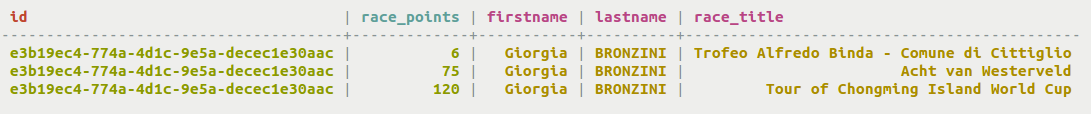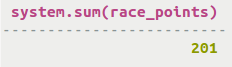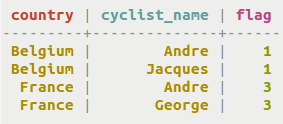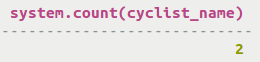# Aggregating results

Using the SELECT command to return data and apply standard aggregate functions.

Starting with DataStax Enterprise 5.0, the standard aggregate functions of `min`, `max`, `avg`, `sum`, and `count` are built-in functions.

## Procedure

• A table cyclist_points records the race points for cyclists.
` CREATE TABLE cycling.cyclist_points (id UUID, firstname text, lastname text, race_title text, race_points int, PRIMARY KEY (id, race_points );`• Calculate the standard aggregation function `sum` to find the sum of race points for a particular cyclist. The value of the aggregate will be returned.
` SELECT sum(race_points) FROM cycling.cyclist_points WHERE id=e3b19ec4-774a-4d1c-9e5a-decec1e30aac;`• Another standard aggregate function is `count`. A table country_flag records the country of each cyclist.
``CREATE TABLE cycling.country_flag (country text, cyclist_name text, flag int STATIC, PRIMARY KEY (country, cyclist_name));``• Calculate the standard aggregation function `count` to find the number of cyclists from Belgium. The value of the aggregate will be returned.
` SELECT count(cyclist_name) FROM cycling.country_flag WHERE country='Belgium';`